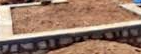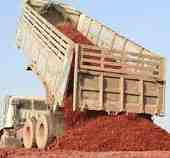### Calculating quantity of construction soil (moorum) required for the plinth filling.

Now, let us calculate the quantity of soil (moorum) required for the plinth filling procedure in the building plan given below.

Here, the depth of the plinth D =750mm.

Deducting rubble soling thickness, which is given as 230mm, we will get soil filling depth

d = [750mm - 230mm.]

= 520mm.= 0.52 mtr.

Now, from the drawing, the filling length

L = (5m.- width of the plinth beam)

=(5- 0.23)

= 4.77mtr.

The width of the filling

W = (3m - width of the plinth beam.)

=(3 - 0.23)

= 2.77mtr.

The volume of the plinth which should be filled by the moorum

= 2×(d ×L ×W)

= 2 × (0.52 × 4.77 × 2.77)

= 13.74 cubic mtr.

Here, we multiply the vol. by 2 for the two identical room plinths, as shown in the drawing.

When we fill the moorum in the plinth and compact them, we need 30% extra material as the moorum supplied to the site are loose soils with voids.

So, the volume of the construction soil (moorum) required

V = 13.74+( (30/100) × 13.74)

= 13.74 + 4.122

=   17.862 cu.mtr.

or compacted soil = [1.3 × loose soil.]

So, the quantity of construction soil required for the plinth filling

= 1.3 × plinth volume

= [1.3 × 13.74]

= 17.862 cum.

To convert it into cubic ft., we will multiply it by 35.315

= [17.862 × 35.315]

=  630.80 cuft. or 6.31 brassPlinth filled with moorum.

Note:  Compacted volume of the soil varies from 1.25 to 1.35 times the loose soil, depending upon the texture and the grain size. But for the calculation purpose, we generally consider it as 1.3 times the loose soil.

### Calculating the number of trucks or tractor trolleys of construction soil required for the plinth filling:

Usually, the container size of the truck is 5cum. by volume. and tractor trolley is of  2cum in volume.Truck dumping the soil.

Now, the number of trucks needed to fill the plinth

= 17.862/5 =      3.57 nos.

The number of tractor trolley required to fill the plinth

= 17.862/2 =  8.931 nos.

or in another way,  suppose if the vol. of a truck container is 1.75 brass and the tractor-trolley is 0.70 brass,

then, the no. of trucks required

= 6.31÷1.75 = 3.60 nos.

The no. of tractor trolley required

= 6.31÷0.70   =  9 nos.

Thank you for going through this calculation😃.

If you have any queries, you can ask me in the comment section.

#### 1 comment:

1.is there any IRC guidilines for srinkage percentage after compaction in roads